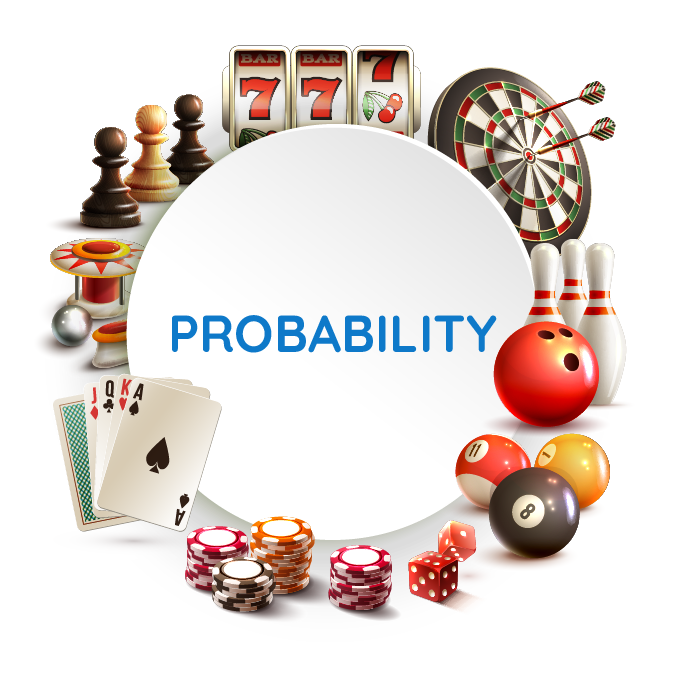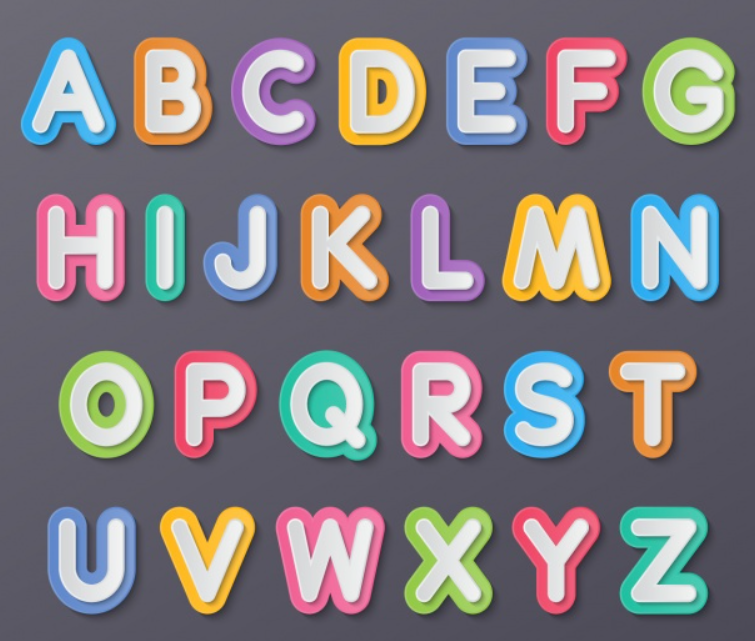# Terms in Probability

Terms in Probability

For games without clear outcomes, probability is helpful to make the best predictions.In this mini-lesson, we shall explore the topic of probability, by knowing the definition of probability, the important terms in probability, by solving the examples and the interactive problems.

## Lesson Plan

 1 What Is Probability? 2 Tips and Tricks 3 Solved Examples 4 Challenging Questions 5 Interactive Questions

## What Is Probability?

### Definition of Probability

The measurement of the possibility of an event is called probability. Since many events cannot be predicted with absolute certainty, probability helps to predict the likelihood of an event to occur. It is the ratio of the number of favorable events to the total number of events in an experiment.

$\text{Probability(Event)} = \dfrac{\text{Favorable Outcomes}}{\text{Total Outcomes}}$

Numerically the probability value always lies between 0 and 1.

$0 \le P(E) \le 1$

It is expressed in percentage, decimal, or fraction.

### Examples

• Probability of getting a head, on tossing a two-faced coin. \begin{align}P(\text{Head} )&= \dfrac{\text{Number of Heads}}{\text{Total Outcomes}} \\&= \dfrac{1}{2} \end{align}
• The probability of getting an odd number, on rolling a six-faced dice.\begin{align}P(\text{Odd Number} )&= \dfrac{\text{Number of odd numbers}}{\text{Total Numbers}} \\&= \dfrac{3}{6} \\&= \dfrac{1}{2} \end{align}
The possibility of picking a diamond card from a well-shuffled pack of cards.\begin{align}P(\text{Diamond Card} )&= \dfrac{\text{No of diamond cards}}{\text{Total cards}}\\& = \dfrac{13}{52}\\& = \dfrac{1}{4}\end{align}Tips and Tricks
1. Probabilities are expressed in fractions, decimals, percentages.
2. Probabilities cannot have negative values.
3. Probability of 1 refers to a sure event and a probability of 0 refers to an impossible event.
4. Probabilities can be found only for clearly defined events.

## What Are the Terms Related to Probability?

The list of the terms related to probability is as follows.

• Experiment: An activity whose outcomes are not known is an experiment. Every experiment has a few favorable outcomes and a few unfavorable outcomes. The historic experiments of Thomas Alva Edison had more than a thousand unsuccessful attempts before he could make a successful attempt to invent the light bulb.
• Random Experiment: A random experiment is an experiment for which the set of possible outcomes is known, but which particular outcome will occur on a particular execution of the experiment cannot be said prior to performing the experiment. Tossing a coin, rolling a die, and drawing a card from a deck are all examples of random experiments.
• Trial: The numerous attempts in the process of an experiment are called trials. In other words, any particular performance of a random experiment is called a trial. For example, tossing a coin is a trial.
• Event: A trial with a clearly defined outcome is an event. For example, getting a tail when tossing a coin is termed as an event.
• Random Event: An event that cannot be easily predicted is a random event.  For such events, the probability value is very less.The formation of a rainbow during the rain is a random event.
• Outcome: This is the result of a trial. In the process of a sportsperson hitting a ball towards the goal post, there are two clear outcomes. He may either make the goal or miss the goal.
• Possible Outcome: The list of all the outcomes in an experiment can be referred to as possible outcomes. In tossing a coin, the possible outcomes are heads or tails.
• Equally likely Outcomes: An experiment in which each of the outcomes has an equal probability, such outcomes are referred to as equally likely outcomes. In the process of rolling a six-faced dice, the probability of getting any number is equal.  $P\text{(any number)} =\dfrac{1}{6}$
• Sample Space:  It is the set of outcomes of all the trials in an experiment. On rolling a dice, the possible outcomes are 1, 2, 3, 4, 5, and 6. These outcomes make up the sample space. S = {1, 2, 3, 4, 5, 6}
• Probable Event: An event that can be predicted is called a probable event. We can calculate the probability of such events. The probability of a particular child being promoted to the next class can be calculated, hence, we can refer to this as a probable event.
• Impossible Event:  An event that is not a part of the experiment, or which does not belong to the sample space of the outcomes of the experiment can be referred to as an impossible event. There is no snowfall in a temperate climatic region.  Here, the snowfall can be referred to as an impossible event because the probability of occurrence of such an event is zero.
• Complementary Events: Complementary events occur when there are just two outcomes, and one event is exactly opposite to another event. For an event with probability $$P(A)$$, its compliment is $$P(\bar A)$$.  $P(A) +P(\bar A) = 1$ In an examination, the event of success and the event of failure are complementary events.  $P(Success) +P(\bar{Failure}) = 1$
• Mutually Exclusive Events: Two events such that the happening of one event prevents the happening of another event are referred to as mutually exclusive events. In other words, two events are said to be mutually exclusive events, if they cannot occur at the same time. For example, tossing a coin can result in either heads or tails. Both cannot be seen at the same time.

## Solved Examples

 Example 1

A football team plays 120 matches and wins 80 matches. What is the probability of the team winning the next match?Solution

Total number of matches played = 120

Number of matches won by the team = 80

\begin{align} \text{Probability of winning} &= \dfrac{\text{Number of matches won}}{\text{Total number of matches}}\\&=\dfrac{80}{120}\\&=\dfrac{2}{3}\end{align}

 $$\therefore$$ The probability is $$\dfrac{2}{3}$$
 Example 2

Tom's teacher wrote each English alphabet on a different piece of paper and jumbled all those in a box. He asked Tom to randomly pick a piece of paper. What is the probability of having a vowel written on that piece of paper?Solution

Total number of English alphabet = 26

Number of vowels = 5

\begin{align} \text{Probability of getting a vowel} &= \dfrac{\text{Number of vowels}}{\text{Total number of alphabets}}\\&=\dfrac{5}{26}\end{align}

 $$\therefore$$ The probability is $$\dfrac{5}{26}$$
 Example 3

Sam takes two coins and flips them both at once. What is the probability of getting heads on both the coins?

Solution

Sample space on flipping two coins = {(H, H), (H, T), (T, H), (T, T)}

Total number of outcomes = 4

Favorable outcome of two heads = 1

\begin{align} \text{Probability of getting two heads} &= \dfrac{\text{Number of outcomes with two heads}}{\text{Total number of outcomes}}\\&=\dfrac{1}{4}\end{align}

 $$\therefore$$ The probability is $$\dfrac{1}{4}$$
 Example 4

Mary had a jar containing 8 red balls, 5 blue balls, and 7 green balls. She called one of her friends and asked them to pick a ball from the jar. What is the probability that the ball which is picked is either a red or a blue ball?Solution

Let us take a count of the number of balls in the jar.

Number of red balls = 8

Number of blue balls = 5

Number of green balls = 7

Total number of balls = 20

\begin{align}\text{P(red or a blue ball)}&= \dfrac{\text{No of red balls + No of blue balls}}{\text{Total number of balls}}\\& = \dfrac{8 + 5}{20}\\ &=\dfrac{13}{20}\end{align}

 $$\therefore$$ The probability is $$\dfrac{13}{20}$$
 Example 5

The probability of Sam being selected in a badminton team is 0.3 and the probability of Maddy being selected is 0.5. The probability of both of them being selected in the team is 0.2. What is the probability that either one of them is selected for the team?

Solution

Probability of Sam's selection =$$P(S) = 0.3$$

Probability of Maddy's selection = $$P(M) = 0.5$$

Probability of both of them being selected = $$P(S \cap M ) = 0.2$$

Probability of either of them being selected = $$P(S \cup M) = ?$$

\begin{align} P(S \cup M) &= P(S) + P(M) -P(S \cap M) \\&=0.3 + 0.5 - 0.2 \\&=0.8 - 0.2 \\&=0.6\end{align}

 $$\therefore$$ The probability of either of them being selected is 0.6Challenging Questions

Let us use two dice for our experiment.  Answer the following questions based on the outcomes of rolling these two dice.

1. What is the probability of getting a doublet?
2. What is the probability of getting a sum of 7?
3. What is the probability of getting a sum of at least 10?

## Interactive Questions on Terms in Probability

Here are a few activities for you to practice. Select/Type your answer and click the "Check Answer" button to see the result.

## Let's Summarize

The mini-lesson targeted the fascinating concept of probability. The math journey around terms in probability starts with what a student already knows, and goes on to creatively crafting a fresh concept in the young minds. Done in a way that not only it is relatable and easy to grasp, but also will stay with them forever. Here lies the magic with Cuemath.

At Cuemath, our team of math experts is dedicated to making learning fun for our favorite readers, the students!

Through an interactive and engaging learning-teaching-learning approach, the teachers explore all angles of a topic.

Be it worksheets, online classes, doubt sessions, or any other form of relation, it’s the logical thinking and smart learning approach that we, at Cuemath, believe in.

## FAQs on Terms in Probability

### 1. What are the three types of probabilities?

The three types of probabilities are :

• Theoretical Probability
• Experimental Probability
• Axiomatic Probability

### 2. What are the 5 rules of probability?

The five rules of probability are:

1. The probability value always lies between 0 and 1.  $$0 \le P(A) \le 1$$
2. The sum of the probabilities of all possible outcomes of an experiment is equal to 1.  $$P(A_1) + P(A_2) + P(A_3) .....P(A_n) = 1$$
3. The sum of probability of an event and its complementary event is equal to 1.  $$P(A) + P(\bar A) = 1$$
4. The sum of the probabilities of two events A and B is $$P(A \cup B) = P(A) + P(B) - P(A \cap B)$$
5. The product of the probability of two events A and B is $$P(A) \cdot P(B) = P(A \cap B)$$

### 3. What is the probability of an event?

The probability of an event is the ratio of the number of outcomes specific to the event and the total number of outcomes of the experiment.  $\text{Probability of an event} = \dfrac{\text{Outcomes specific to the event}}{\text{Total outcomes of the experiment}}$

### 4. What is the probability of a sure event?

The probability of a sure event is equal to 1.

### 5. What is the maximum and minimum probability of an event?

The maximum probability of an event is 1 and the minimum probability of an event is 0.

### 6. What are the 3 axioms of probability?

The three axioms of probability are:

1. The probability of an event is always a real number between 0 and 1.
2. The sum of the probabilities of all the events in an experiment is 1.
3. The happening of either of the two independent events is equal to the sum of their individual probabilities. $P(A \cup B) = P(A) + P(B)$

### 7. Can a probability be negative?

The probability value cannot be negative. It is a positive value between 0 and 1.

### 8. What is the formula of probability?

Probability is the ratio of the favorable events to the total events in an experiment.  $Probability = \dfrac{\text{Favourable Events}}{\text{Total number of Events}}$

### 9. Can you have a probability greater than 1?

The probability of a number is never greater than 1. It is always between 0 and 1. $0 \le P(A) \le 1$

### 10. What does the probability of 1 mean?

The probability of 1 means a sure event.

Probability